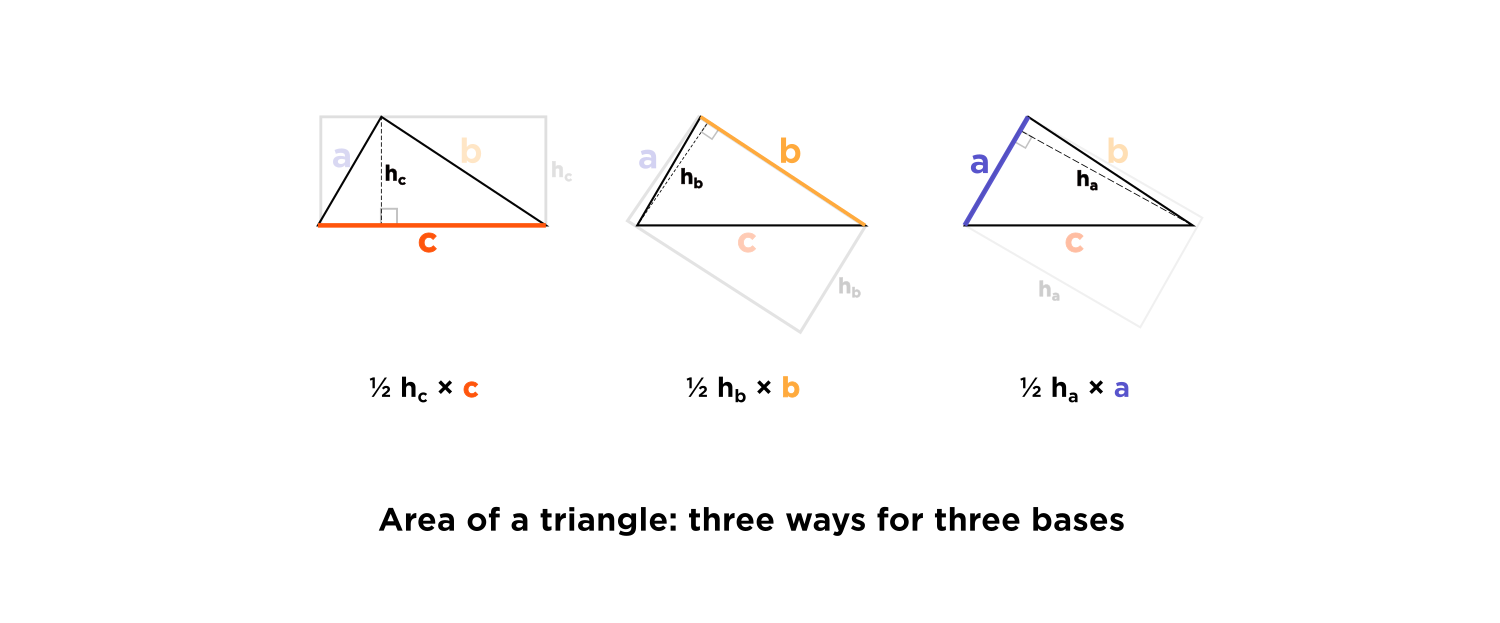# Why isn't the area of a triangle just side × side ÷ 2?

• Someone little today gave me several answers today to the question, "What's the area of this triangle?"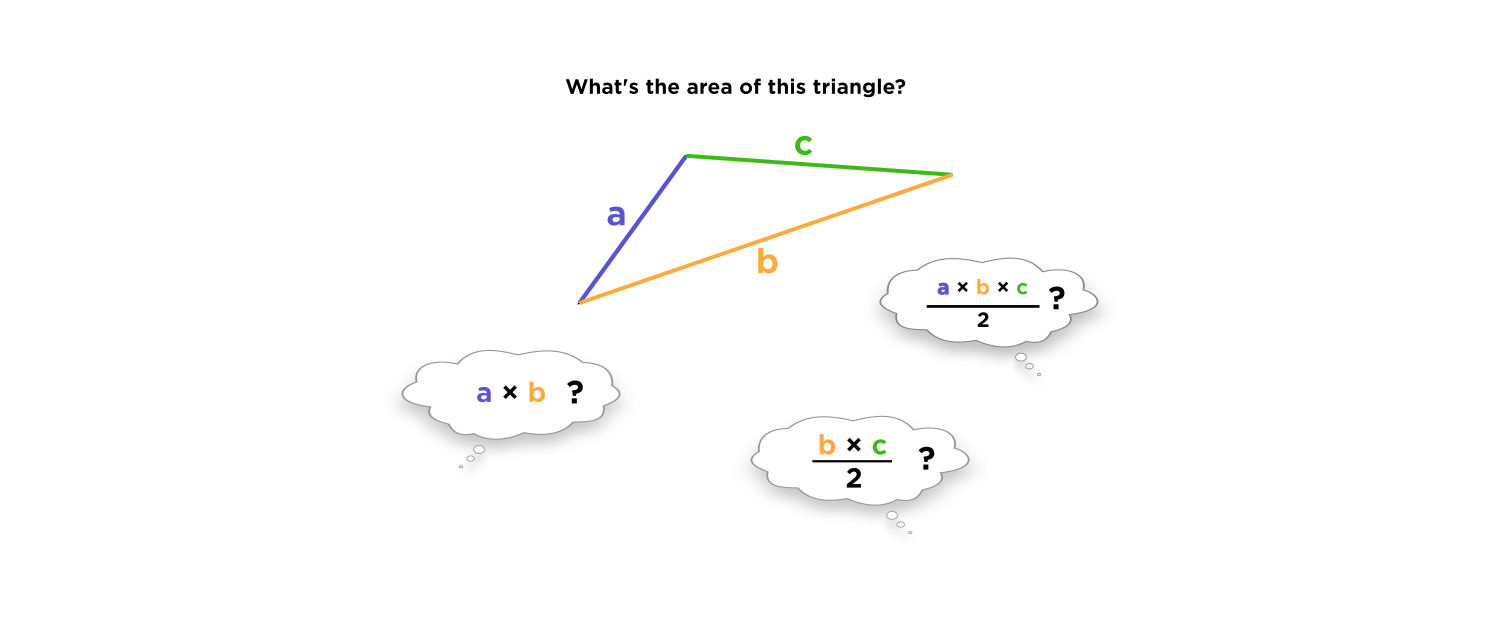At first I was a bit shocked, appalled and dismayed at this answer... However, after thinking about it a while, I guess it's a very natural thing for a little person to say!A triangle looks like it should be $$\color{red}\text{easy}$$ to calculate the area of. It's an easy shape, in the same way that a rectangle or a square is "easy":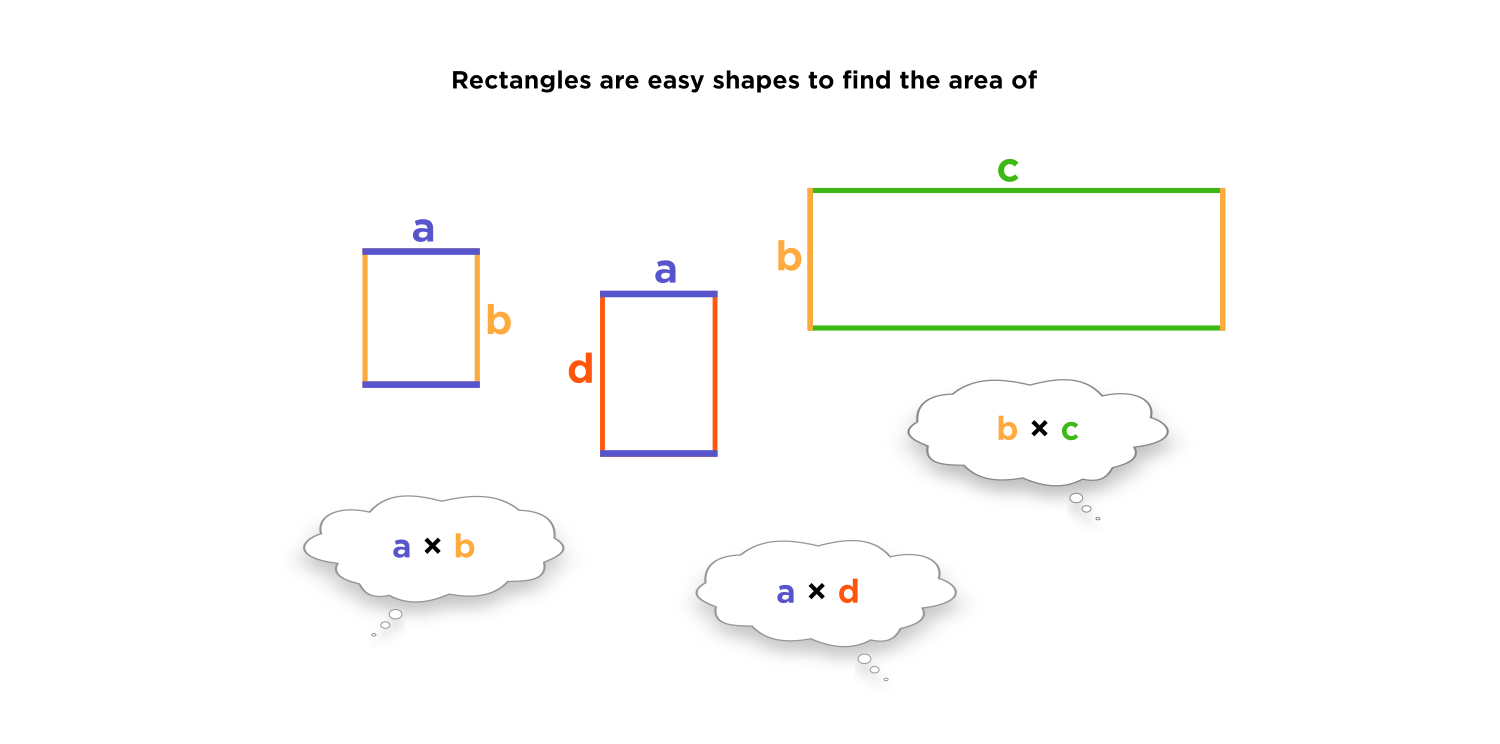But if I could just multiply two of the sides of a triangle to get its area, then what if I draw the triangles in a weird way? What if I draw a very short and skinny triangle, like this?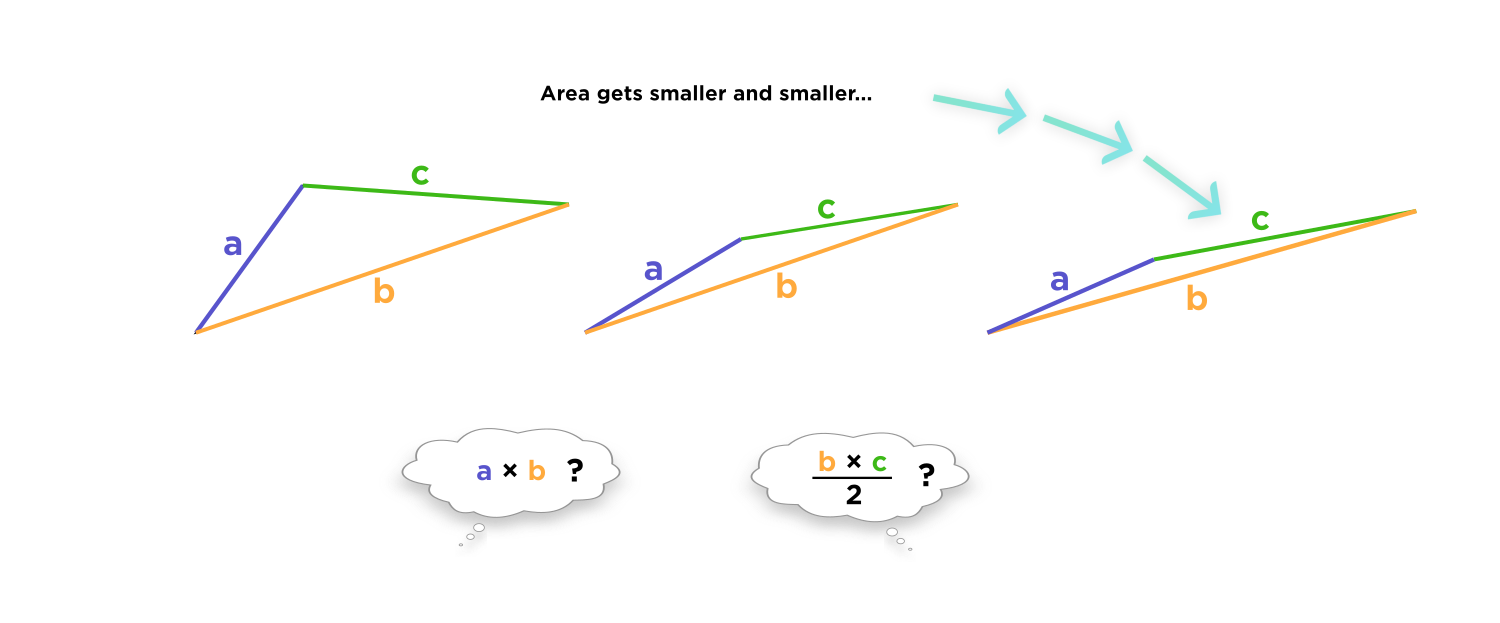Does it really seem like the area of a triangle is related to the product of its sides? I can draw a triangle with the same two sides, but skinnier and skinnier until its area is almost $$0.$$

My friend sort of "remembered" the formula for the area of a triangle, except she remembered the formula for a right triangle's area. She understood that a right triangle is half of the bounding rectangle that surrounds it. But she didn't understand exactly why the formula was true, so had trouble relating it to a non-right triangle.

$$\textcolor{red}{\text{Area of a right triangle}} = \frac{1}{2} \text{ } \text{ base } \times \text{ height}$$

Now, why is the area of the second triangle equal to the first triangle? She cuts the first right triangle and says it's because the two mini-triangles are the same size.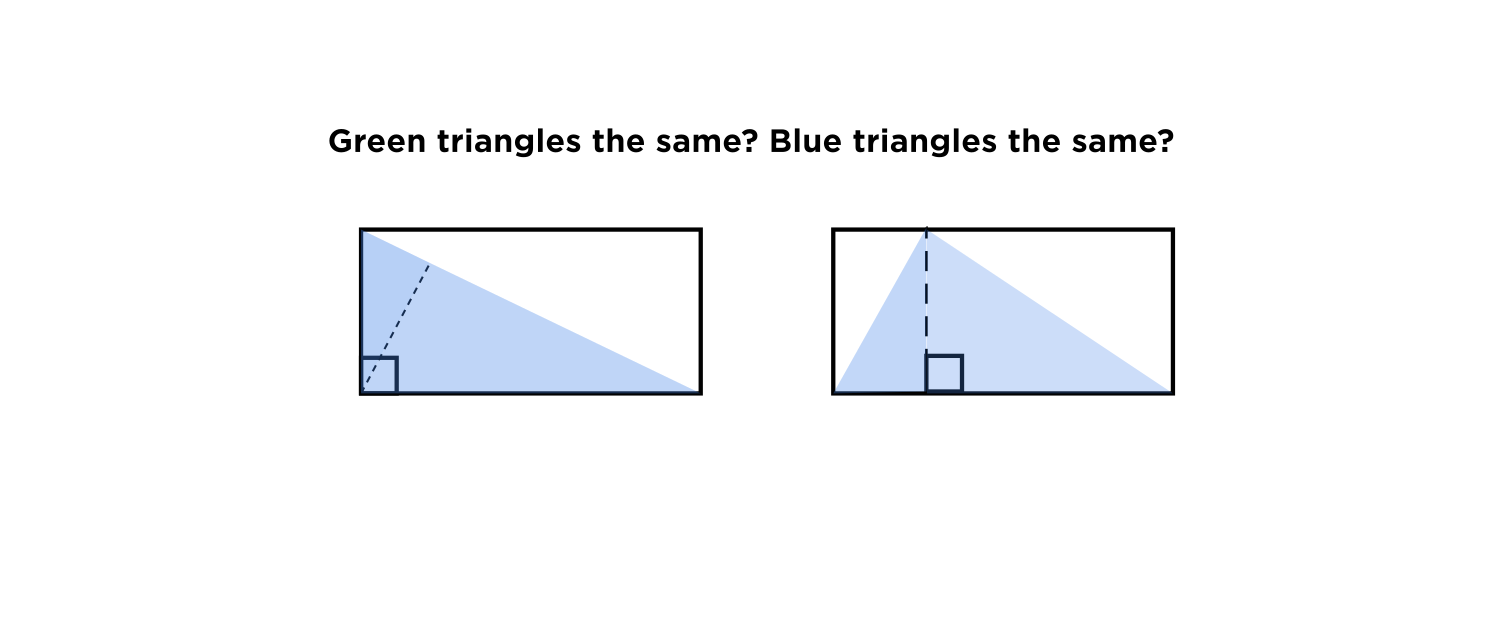Is that really true?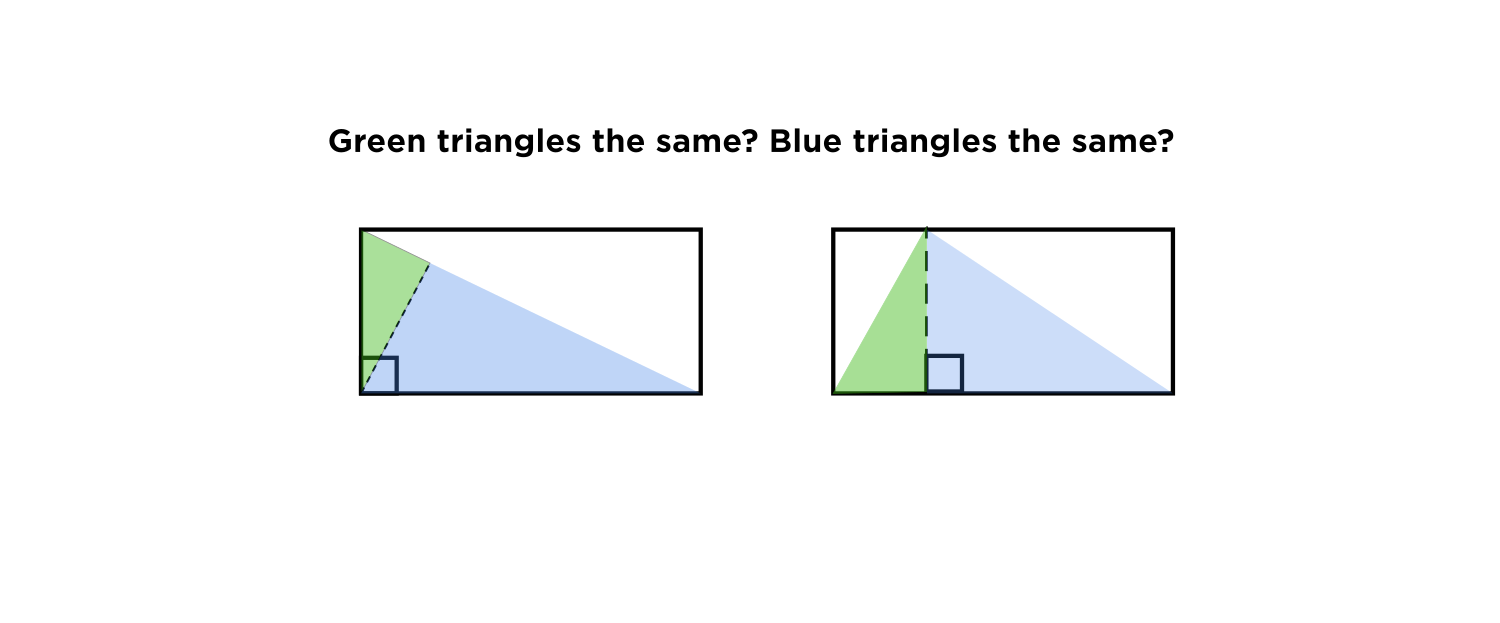No, it's not! They look the same, but their lengths are actually different. The longest side of the left triangle is a diagonal, which is longer than the longest side of the right triangle, which is just the long side of the rectangle. Also, the altitudes (heights) of the triangle are different. The altitude on the left, colored yellow, is shorter than the height of the rectangle, so it is shorter than the altitude of the right triangle.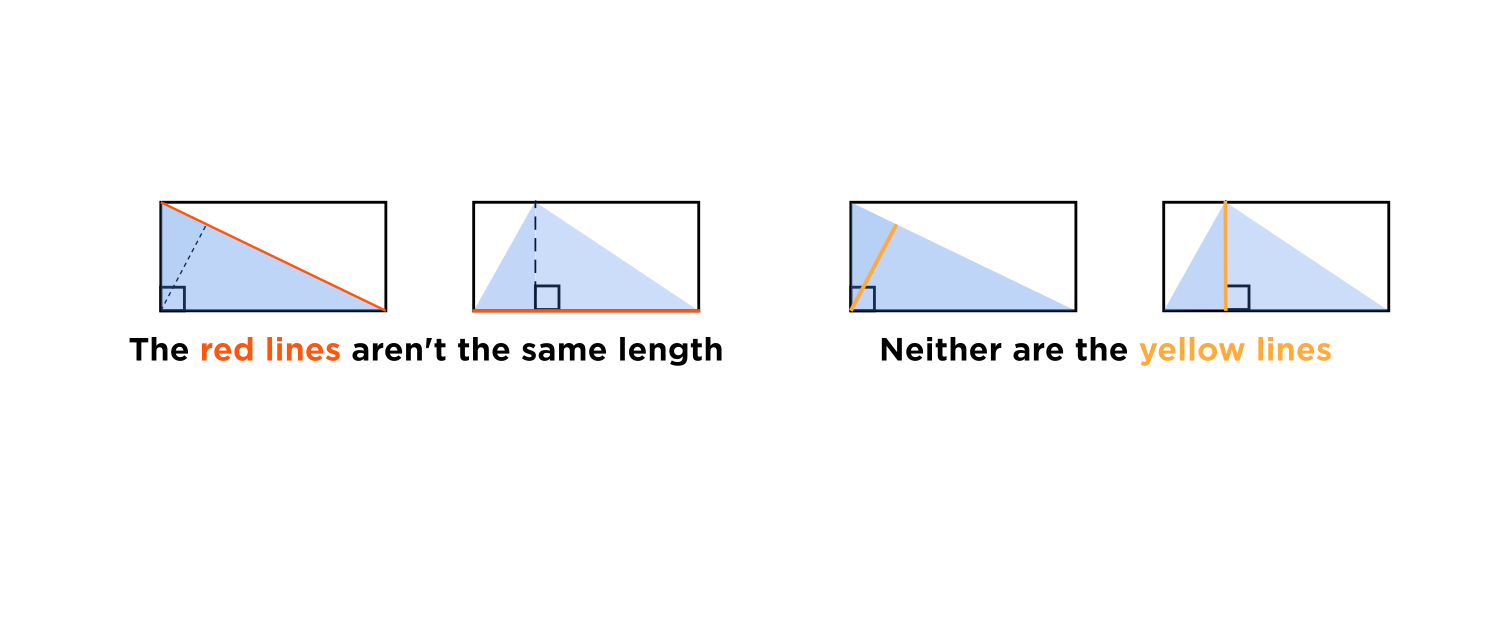There's a different reason why both triangles inscribed in the box have the same area. It's because you can split the box into two smaller boxes, each of which has half taken up by a mini-triangle.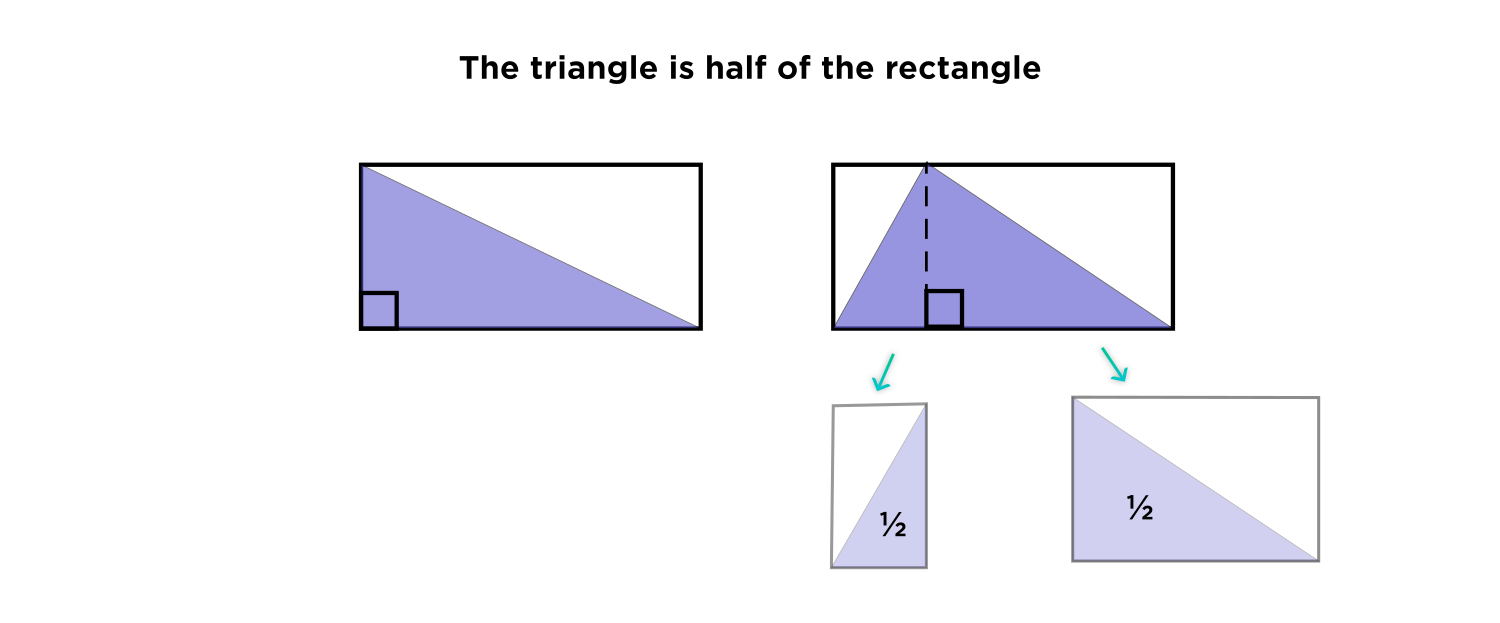Thus we say the area of a triangle is equal to $$\color{red}\frac{1}{2} \times \text{ } \text{ base } \times \text{ height},$$ where the height is the height of the triangle's bounding rectangle.

We can interpret any of the three sides of the triangle as the base; just remember to draw a bounding rectangle and find the height of this rectangle. This altitude is always perpendicular ( $$\perp$$ ) to the base.

Since each of the three sides can be a different base, there are three formulas for the area of a triangle.